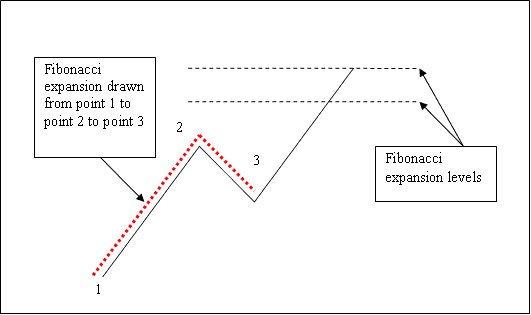# How to Analyze 100.0 Fibonacci Indices Trading Projection Level

We useFibonacci expansionlevels to estimate where the indices trend movement will reach. There are 2 important Fibonacci expansion levels; 61.8% and 100.0% Fibonacci Projection Levels, these are used for taking profit.

## 100.0 Indices Trading Fib Projection Level

100.0 Indices Trading Fib Projection Level is not commonly used to set indices trading profit taking orders when trading using the Fibonacci Projection Indicator.

Most indices traders will use the 61.8% Fibonacci Projection level.

To draw Fibonacci Projection levels on the stock indices chart we wait until the stock indexes price retracement is complete and the stock indexes price starts to move in the original direction of the Indices trend. Where the stock indexes price retracement reaches is used as chart point 3. Fibonacci Projection Indicator is drawn using three chart points as shown on the trading example explained and illustrated below of how to draw the Fibonacci Projection Indicator Tool.

The Fibonacci expansion example explained and illustrated below shows the 3 Chart Points where the Fibonacci expansion indicator is drawn, marked as Chart point 1, Chart point 2 and Chart point 3.

Chart point 1 is where the indices trend started, Chart point 2 is where the indices trend pulled back and retraced and Chart point 3 is where the stock indices retracement reached as shown on the Fibonacci Projection Indicator Tool example explained and illustrated below.

The First Fibonacci Projection Level is the 61.8% Indices Trading Fib Projection Level and the second is 100.0 Indices Trading Fib Projection Level shown Below - These Fibonacci Projection Levels are Used by Indices Traders to Set Take Profit Indices Trading Orders61.8% Indices Trading Fib Projection and 100.0% Indices Trading Fib Projection Level Used to Set Take Profit Indices Trading Orders

Please note where these Fibonacci Projection levels are drawn - Fibonacci Projection levels are drawn above the Fibonacci indicator, these are the chart points where a indices trader will set the take profit orders using these Fibonacci Projection Levels - 100.0% Fibonacci Projection Level is the second Fibonacci Level.